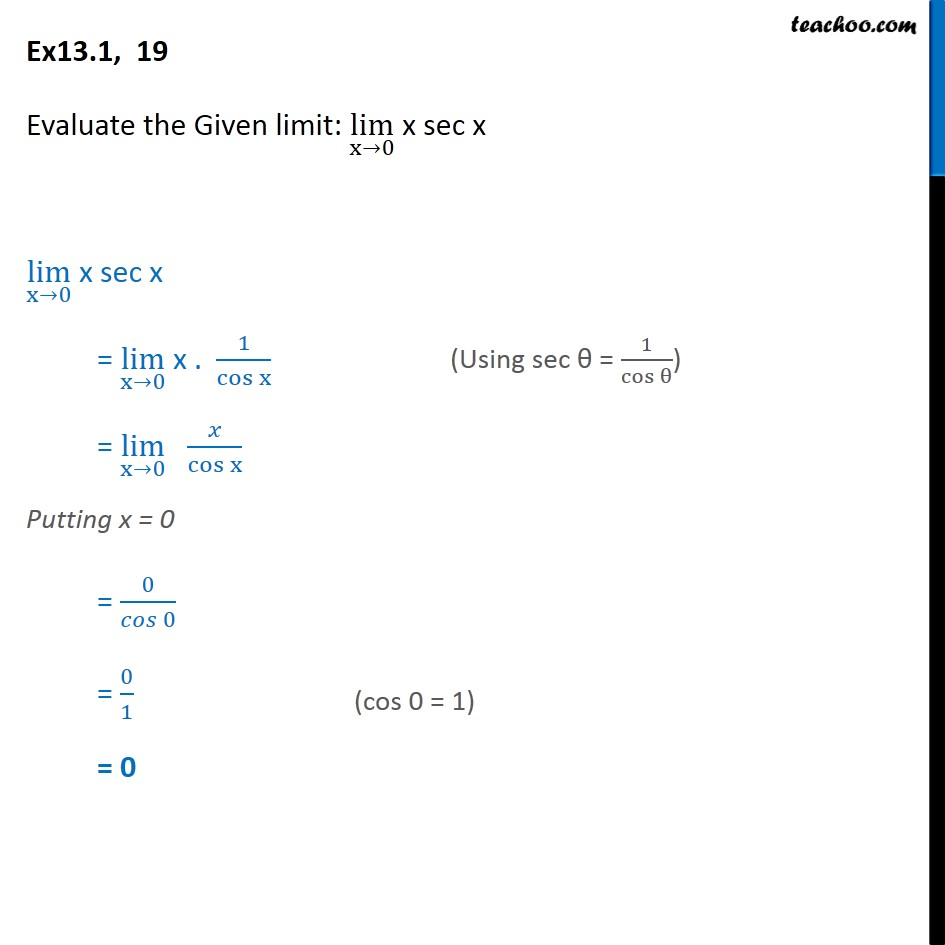1. Chapter 13 Class 11 Limits and Derivatives
2. Serial order wise
3. Ex 13.1

Transcript

Ex 13.1, 19 - Chapter 13 Class 11 Limits and Derivatives - NCERT Evaluate the Given limit: lim (x→0) x sec x lim (x→0) x sec x (Using sec θ = 1/cos⁡θ ) = lim (x→0) x . 1/cos⁡x = lim (x→0) x/cos⁡x Putting x = 0 = 0/(cos 0) = 0/1 (cos 0 = 1) = 0

Ex 13.1